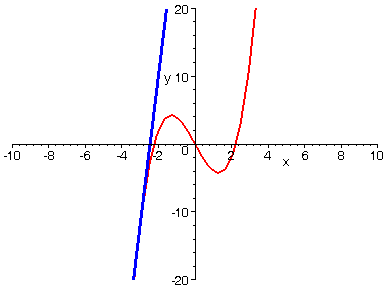ANIMATED TANGENT LINE

This animation shows a tangent line moving along a curve. Some days you've just got way too much time on your hands! Nonetheless, if you contemplate it, you'll be able to visually see what tangent lines have to do with where a function is increasing, decreasing, concavity, inflection points, and where a function has a relative maximum or minimum.

> P:=plot(x^3-5*x, x=-10..10, y=-20..20, thickness=2):

> Q:=animate( (3*t^2-5)*(x-t)+t^3-5*t,x=-10..10,t=-3..3,thickness=3, color=blue,frames=100, numpoints=100):

> display([P,Q]);>